CS 1010 Programming Methodology http www comp nus

• Slides: 55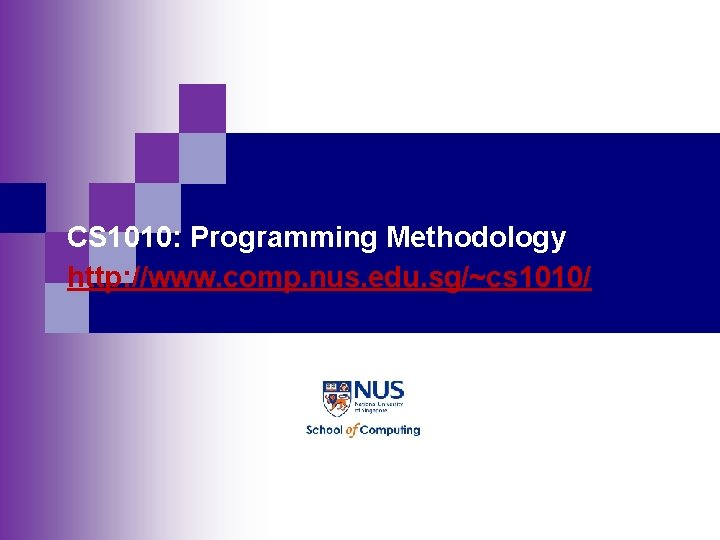CS 1010: Programming Methodology http: //www. comp. nus. edu. sg/~cs 1010/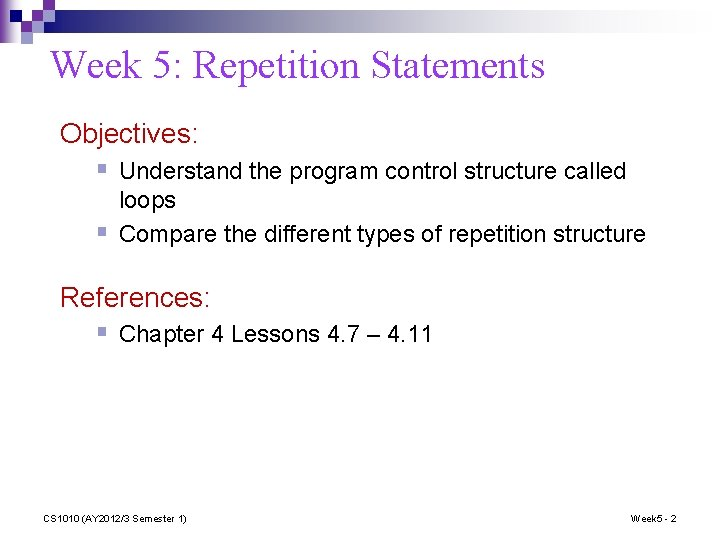Week 5: Repetition Statements Objectives: § Understand the program control structure called § loops Compare the different types of repetition structure References: § Chapter 4 Lessons 4. 7 – 4. 11 CS 1010 (AY 2012/3 Semester 1) Week 5 - 2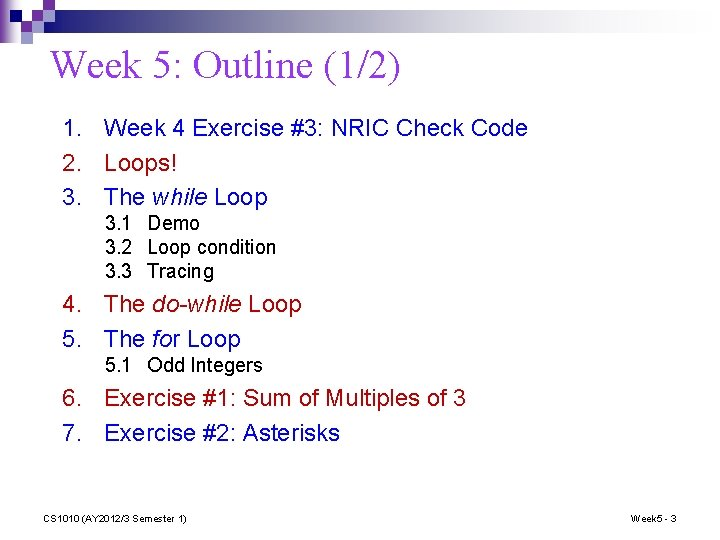Week 5: Outline (1/2) 1. Week 4 Exercise #3: NRIC Check Code 2. Loops! 3. The while Loop 3. 1 Demo 3. 2 Loop condition 3. 3 Tracing 4. The do-while Loop 5. The for Loop 5. 1 Odd Integers 6. Exercise #1: Sum of Multiples of 3 7. Exercise #2: Asterisks CS 1010 (AY 2012/3 Semester 1) Week 5 - 3Week 5: Outline (2/2) 8. Common Errors 9. Some Notes of Caution 10. Exercise #3: Tracing Nested Loops 11. Using break in Loop 12. Using continue in Loop 13. Exercise #4: Prime Number (take-home) CS 1010 (AY 2012/3 Semester 1) Week 5 - 4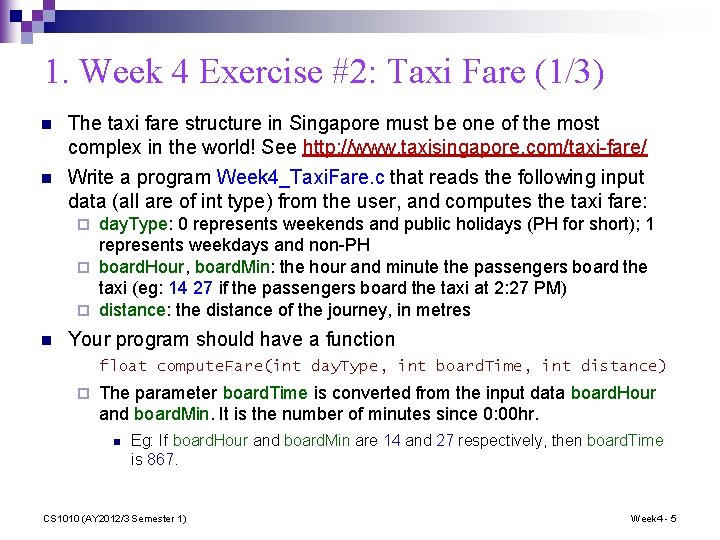1. Week 4 Exercise #2: Taxi Fare (1/3) n The taxi fare structure in Singapore must be one of the most complex in the world! See http: //www. taxisingapore. com/taxi-fare/ n Write a program Week 4_Taxi. Fare. c that reads the following input data (all are of int type) from the user, and computes the taxi fare: day. Type: 0 represents weekends and public holidays (PH for short); 1 represents weekdays and non-PH ¨ board. Hour, board. Min: the hour and minute the passengers board the taxi (eg: 14 27 if the passengers board the taxi at 2: 27 PM) ¨ distance: the distance of the journey, in metres ¨ n Your program should have a function float compute. Fare(int day. Type, int board. Time, int distance) ¨ The parameter board. Time is converted from the input data board. Hour and board. Min. It is the number of minutes since 0: 00 hr. n Eg: If board. Hour and board. Min are 14 and 27 respectively, then board. Time is 867. CS 1010 (AY 2012/3 Semester 1) Week 4 - 5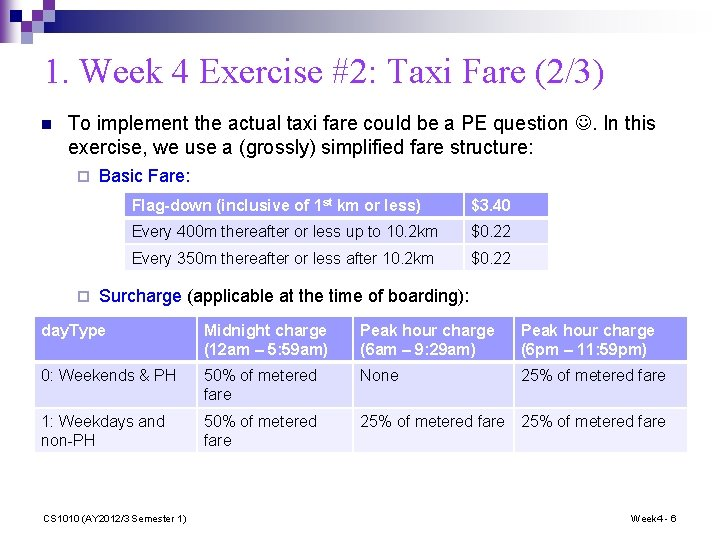1. Week 4 Exercise #2: Taxi Fare (2/3) n To implement the actual taxi fare could be a PE question . In this exercise, we use a (grossly) simplified fare structure: ¨ ¨ Basic Fare: Flag-down (inclusive of 1 st km or less) \$3. 40 Every 400 m thereafter or less up to 10. 2 km \$0. 22 Every 350 m thereafter or less after 10. 2 km \$0. 22 Surcharge (applicable at the time of boarding): day. Type Midnight charge (12 am – 5: 59 am) Peak hour charge (6 am – 9: 29 am) Peak hour charge (6 pm – 11: 59 pm) 0: Weekends & PH 50% of metered fare None 25% of metered fare 1: Weekdays and non-PH 50% of metered fare 25% of metered fare CS 1010 (AY 2012/3 Semester 1) Week 4 - 61. Week 4 Exercise #2: Taxi Fare (3/3) n You are given an incomplete program Week 4_Taxi. Fare. Partial. c. Complete the program. This exercise is mounted on Code. Crunch. n Sample runs below for your checking Day type: 0 Boarding hour and minute: 14 27 Distance: 10950 Total taxi fare is \$9. 12 First 1 km: \$3. 40 Next 9. 2 km: 23 \$0. 22 = \$5. 06 Next 750 m: 3 \$0. 22 = \$0. 66 Basic fare = \$9. 12 No surcharge Total fare = \$9. 12 Day type: 1 Boarding hour and minute: 9 20 Distance: 6123 Total taxi fare is \$7. 83 First 1 km: \$3. 40 Next 5123 m: 13 \$0. 22 = \$2. 86 Basic fare = \$6. 26 Surcharge = 25% \$6. 26 = \$1. 57 Total fare = \$7. 83 Day type: 1 Boarding hour and minute: 5 59 Distance: 9000 Total taxi fare is \$11. 70 First 1 km: \$3. 40 Next 8 km: 20 \$0. 22 = \$4. 40 Basic fare = \$7. 80 Surcharge = 50% \$7. 80 = \$3. 90 Total fare = \$11. 70 CS 1010 (AY 2012/3 Semester 1) Week 4 - 71. Week 4 Exercise #2: Taxi Fare float compute. Fare(int daytype, int b. Time, int dist) { basic. Fare = calc. Basic. Fare(dist) ; return include. Surcharge(basic. Fare, daytype, b. Time) ; } float calc. Basic. Fare(int dist){ // Pre-cond: 0 <= dist ; if (dist <= 1000) return 3. 40; else if (dist <= 10200 && dist > 1000) return (3. 40 + ceil((dist - 1000) /400. 0) * INCREMENT); else if (dist > 10200) return (3. 40 + 9200 / 400. 0 * INCREMENT + (ceil((dist - 10200) / 350. 0)) * INCREMENT); } CS 1010 (AY 2012/3 Semester 1) Week 4 - 8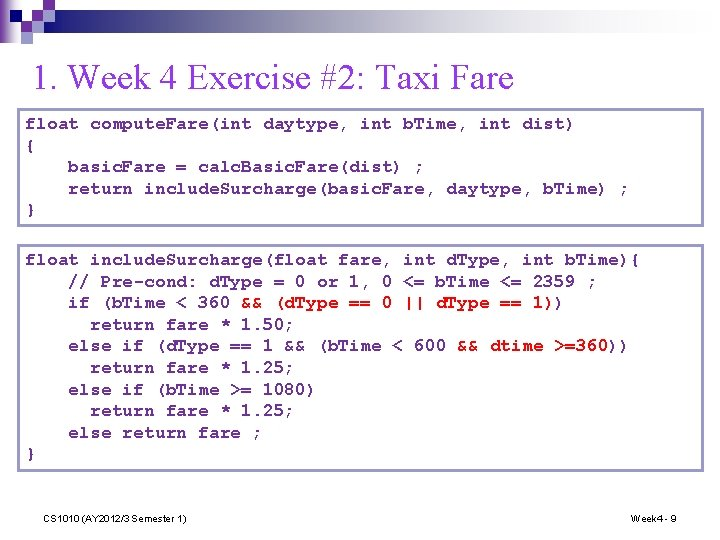1. Week 4 Exercise #2: Taxi Fare float compute. Fare(int daytype, int b. Time, int dist) { basic. Fare = calc. Basic. Fare(dist) ; return include. Surcharge(basic. Fare, daytype, b. Time) ; } float include. Surcharge(float fare, int d. Type, int b. Time){ // Pre-cond: d. Type = 0 or 1, 0 <= b. Time <= 2359 ; if (b. Time < 360 && (d. Type == 0 || d. Type == 1)) return fare * 1. 50; else if (d. Type == 1 && (b. Time < 600 && dtime >=360)) return fare * 1. 25; else if (b. Time >= 1080) return fare * 1. 25; else return fare ; } CS 1010 (AY 2012/3 Semester 1) Week 4 - 9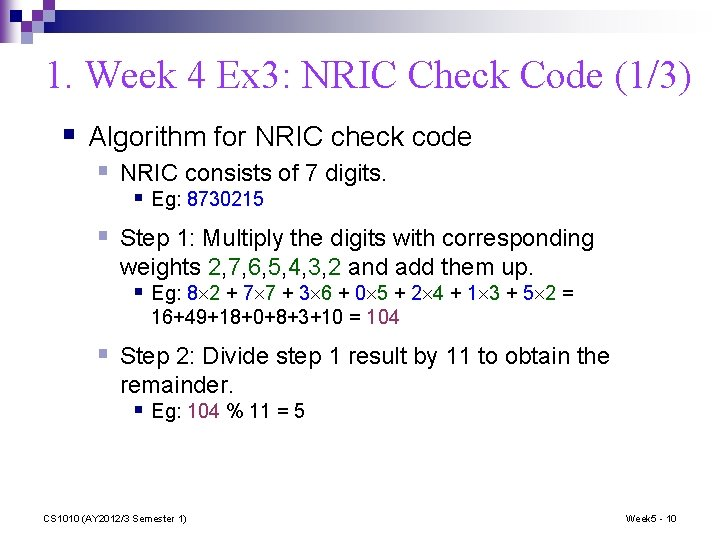1. Week 4 Ex 3: NRIC Check Code (1/3) § Algorithm for NRIC check code § NRIC consists of 7 digits. § Eg: 8730215 § Step 1: Multiply the digits with corresponding weights 2, 7, 6, 5, 4, 3, 2 and add them up. § Eg: 8 2 + 7 7 + 3 6 + 0 5 + 2 4 + 1 3 + 5 2 = 16+49+18+0+8+3+10 = 104 § Step 2: Divide step 1 result by 11 to obtain the remainder. § Eg: 104 % 11 = 5 CS 1010 (AY 2012/3 Semester 1) Week 5 - 101. Week 4 Ex 3: NRIC Check Code (2/3) § Algorithm for NRIC check code (cont…) § Step 3: Subtract step 2 result from 11 § Eg: 11 – 5 = 6 § Step 4: Match step 3 result in this table for the check code 1 2 3 4 5 6 7 8 9 10 11 A B C D E F G H I Z J § Eg: The check code corresponding to 6 is ‘F’. § Therefore, the check code for 8730215 is ‘F’. § Sample run: CS 1010 (AY 2012/3 Semester 1) Enter 7 -digit NRIC number: 8730215 Check code is F Week 5 - 11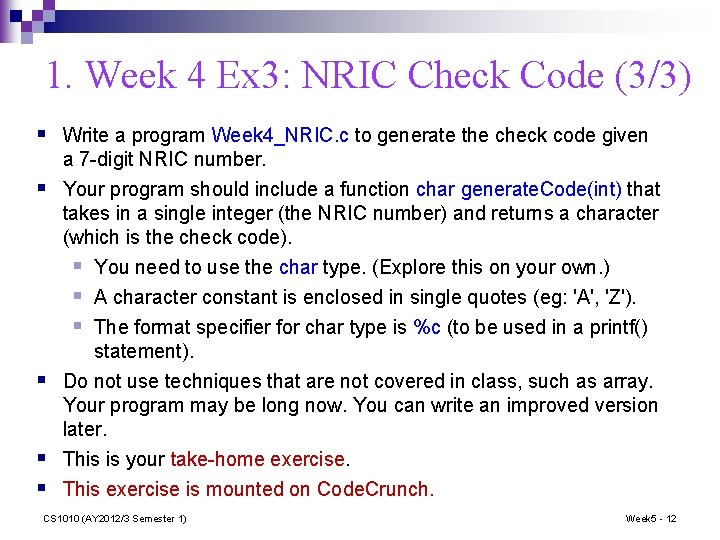1. Week 4 Ex 3: NRIC Check Code (3/3) § Write a program Week 4_NRIC. c to generate the check code given a 7 -digit NRIC number. § Your program should include a function char generate. Code(int) that § § § takes in a single integer (the NRIC number) and returns a character (which is the check code). § You need to use the char type. (Explore this on your own. ) § A character constant is enclosed in single quotes (eg: 'A', 'Z'). § The format specifier for char type is %c (to be used in a printf() statement). Do not use techniques that are not covered in class, such as array. Your program may be long now. You can write an improved version later. This is your take-home exercise. This exercise is mounted on Code. Crunch. CS 1010 (AY 2012/3 Semester 1) Week 5 - 121. Week 4 Ex 3: NRIC Check code § Step 1: Multiply the digits with corresponding weights 2, 7, 6, 5, 4, 3, 2 and add them up. § Eg: 8 2 + 7 7 + 3 6 + 0 5 + 2 4 + 1 3 + 5 2 = 16+49+18+0+8+3+10 = 104 // Extract the digits digit 7 = num%10; num /= 10; digit 6 = num%10; num /= 10; digit 5 = num%10; num /= 10; digit 4 = num%10; num /= 10; digit 3 = num%10; num /= 10; digit 2 = num%10; num /= 10; digit 1 = num%10; step 1 = digit 1*2 + digit 2*7 + digit 3*6 + digit 4*5 + digit 5*4 + digit 6*3 + digit 7*2; Week 5 - 13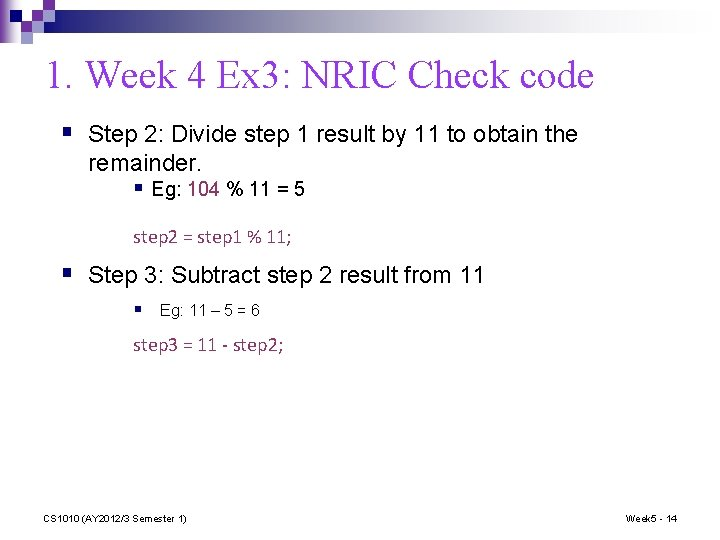1. Week 4 Ex 3: NRIC Check code § Step 2: Divide step 1 result by 11 to obtain the remainder. § Eg: 104 % 11 = 5 step 2 = step 1 % 11; § Step 3: Subtract step 2 result from 11 § Eg: 11 – 5 = 6 step 3 = 11 - step 2; CS 1010 (AY 2012/3 Semester 1) Week 5 - 14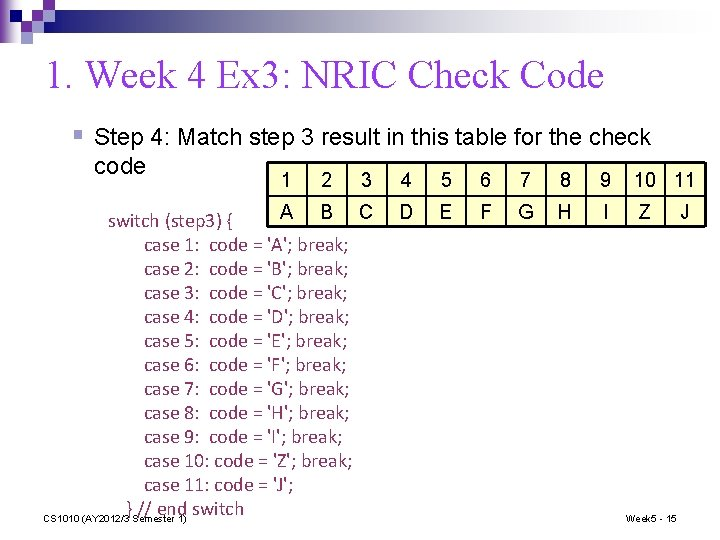1. Week 4 Ex 3: NRIC Check Code § Step 4: Match step 3 result in this table for the check code 1 2 3 4 5 6 7 8 9 10 11 A B C switch (step 3) { case 1: code = 'A'; break; case 2: code = 'B'; break; case 3: code = 'C'; break; case 4: code = 'D'; break; case 5: code = 'E'; break; case 6: code = 'F'; break; case 7: code = 'G'; break; case 8: code = 'H'; break; case 9: code = 'I'; break; case 10: code = 'Z'; break; case 11: code = 'J'; } // end switch CS 1010 (AY 2012/3 Semester 1) D E F G H I Z Week 5 - 15 JRecall: Control Structures Sequence Selection if-else, switch Repetition CS 1010 (AY 2012/3 Semester 1) Week 5 - 16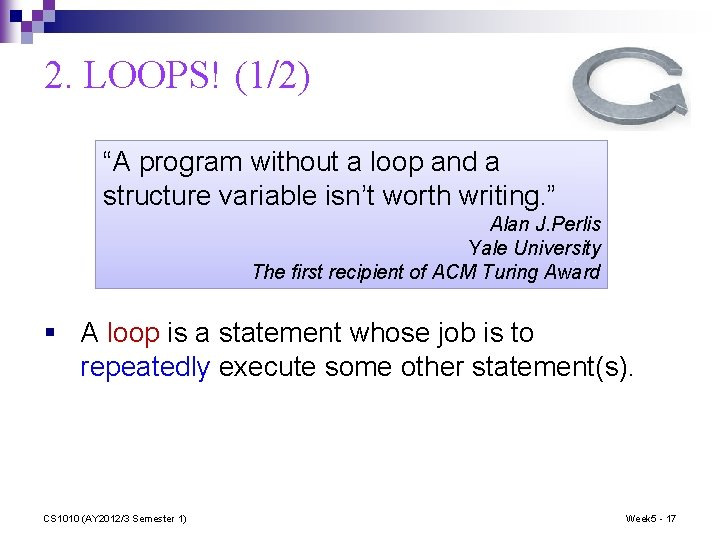2. LOOPS! (1/2) “A program without a loop and a structure variable isn’t worth writing. ” Alan J. Perlis Yale University The first recipient of ACM Turing Award § A loop is a statement whose job is to repeatedly execute some other statement(s). CS 1010 (AY 2012/3 Semester 1) Week 5 - 17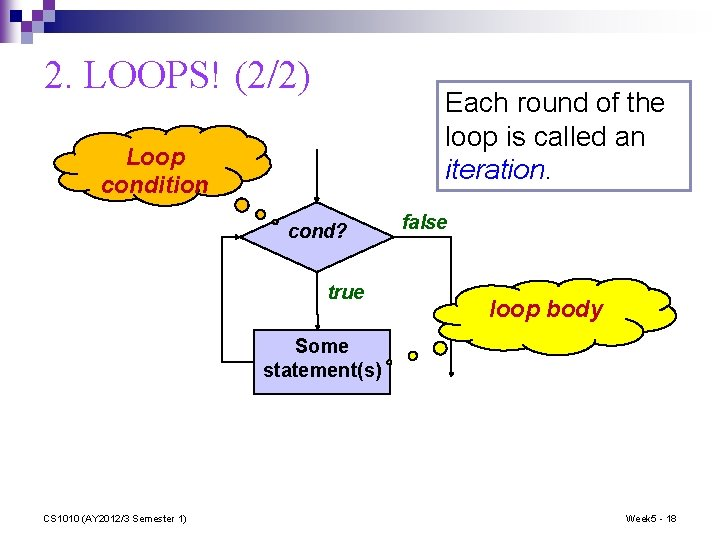2. LOOPS! (2/2) Each round of the loop is called an iteration. Loop condition cond? true false loop body Some statement(s) CS 1010 (AY 2012/3 Semester 1) Week 5 - 18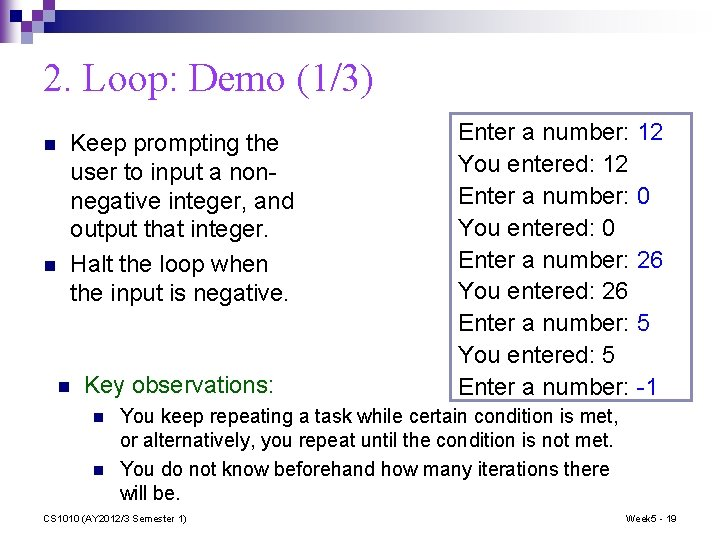2. Loop: Demo (1/3) n n Keep prompting the user to input a nonnegative integer, and output that integer. Halt the loop when the input is negative. n Key observations: n n Enter a number: 12 You entered: 12 Enter a number: 0 You entered: 0 Enter a number: 26 You entered: 26 Enter a number: 5 You entered: 5 Enter a number: -1 You keep repeating a task while certain condition is met, or alternatively, you repeat until the condition is not met. You do not know beforehand how many iterations there will be. CS 1010 (AY 2012/3 Semester 1) Week 5 - 19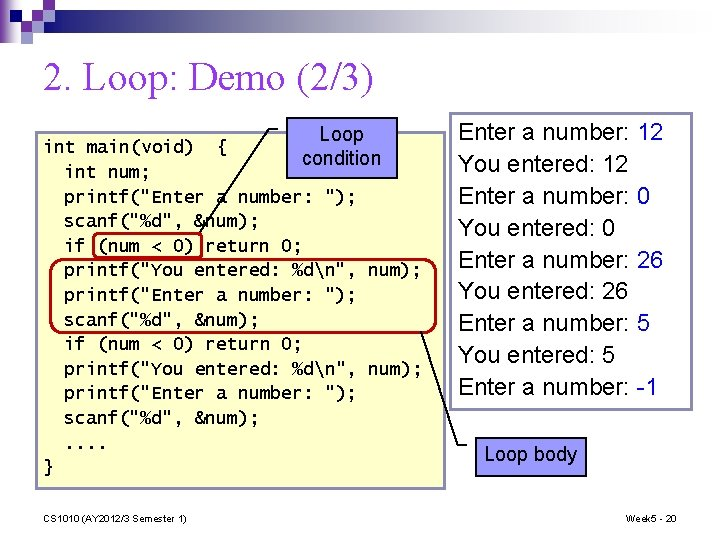2. Loop: Demo (2/3) Loop int main(void) { condition int num; printf("Enter a number: "); scanf("%d", &num); if (num < 0) return 0; printf("You entered: %dn", num); printf("Enter a number: "); scanf("%d", &num); . . } CS 1010 (AY 2012/3 Semester 1) Enter a number: 12 You entered: 12 Enter a number: 0 You entered: 0 Enter a number: 26 You entered: 26 Enter a number: 5 You entered: 5 Enter a number: -1 Loop body Week 5 - 20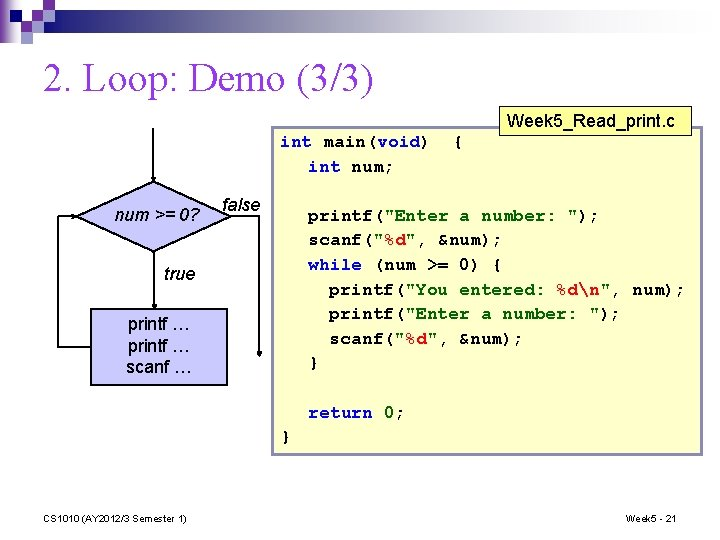2. Loop: Demo (3/3) Week 5_Read_print. c int main(void) int num; num >= 0? false { printf("Enter a number: "); scanf("%d", &num); while (num >= 0) { printf("You entered: %dn", num); printf("Enter a number: "); scanf("%d", &num); } true printf … scanf … return 0; } CS 1010 (AY 2012/3 Semester 1) Week 5 - 21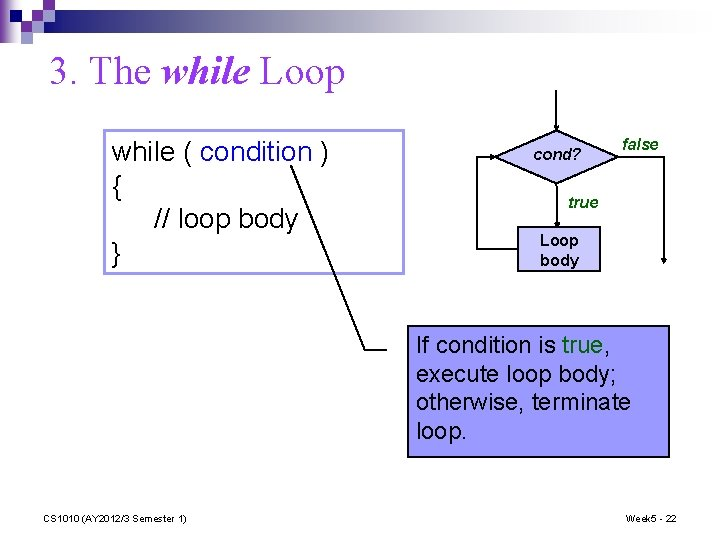3. The while Loop while ( condition ) { // loop body } cond? false true Loop body If condition is true, execute loop body; otherwise, terminate loop. CS 1010 (AY 2012/3 Semester 1) Week 5 - 22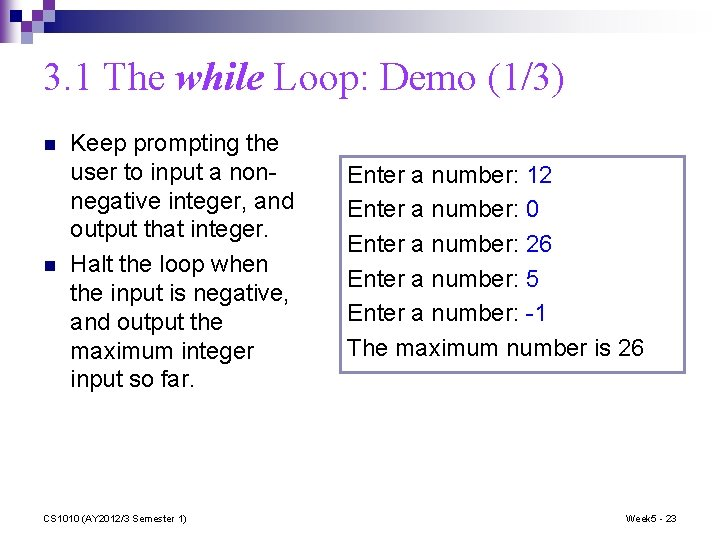3. 1 The while Loop: Demo (1/3) n n Keep prompting the user to input a nonnegative integer, and output that integer. Halt the loop when the input is negative, and output the maximum integer input so far. CS 1010 (AY 2012/3 Semester 1) Enter a number: 12 Enter a number: 0 Enter a number: 26 Enter a number: 5 Enter a number: -1 The maximum number is 26 Week 5 - 233. 1 The while Loop: Demo (2/3) maxi = 0; num input; if (num < 0) { print maxi; stop; } if (maxi < num) maxi = num; num input; . . . CS 1010 (AY 2012/3 Semester 1) maxi = 0; num input; while (num >= 0) { if (maxi < num) maxi = num; num input; } print maxi; Week 5 - 24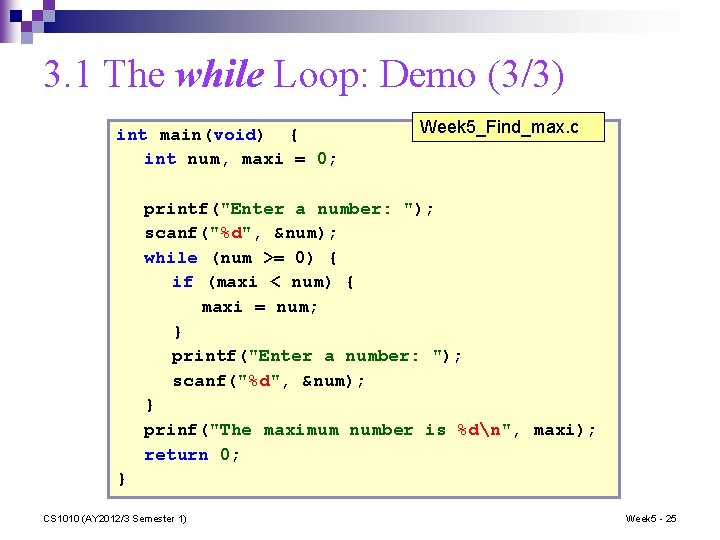3. 1 The while Loop: Demo (3/3) int main(void) { int num, maxi = 0; Week 5_Find_max. c printf("Enter a number: "); scanf("%d", &num); while (num >= 0) { if (maxi < num) { maxi = num; } printf("Enter a number: "); scanf("%d", &num); } prinf("The maximum number is %dn", maxi); return 0; } CS 1010 (AY 2012/3 Semester 1) Week 5 - 253. 2 while Loop Condition (1/2) a = 2; Output: ? b = 7; while (a == b) { print a; a = a + 2; } § When the loop condition is always false, the loop body is not executed. CS 1010 (AY 2012/3 Semester 1) Week 5 - 263. 2 while Loop Condition (2/2) a = 2; Output: ? b = 7; while (a != b) { print a; a = a + 2; } CS 1010 (AY 2012/3 Semester 1) Week 5 - 27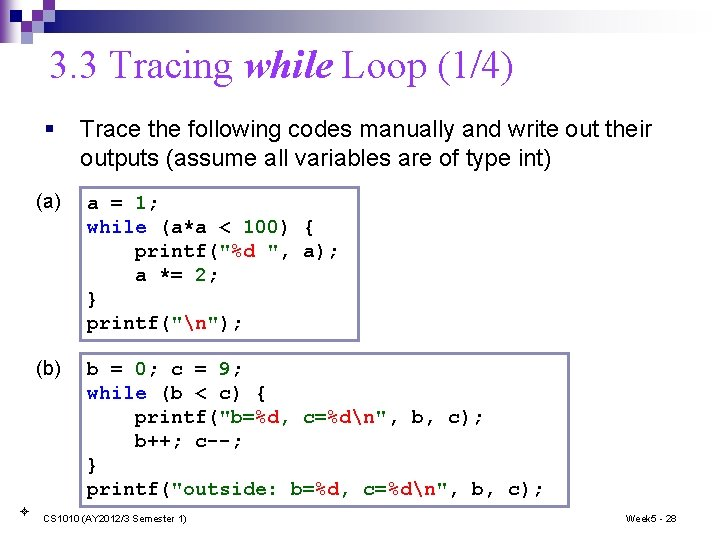3. 3 Tracing while Loop (1/4) § Trace the following codes manually and write out their outputs (assume all variables are of type int) (a) a = 1; while (a*a < 100) { printf("%d ", a); a *= 2; } printf("n"); (b) b = 0; c = 9; while (b < c) { printf("b=%d, c=%dn", b, c); b++; c--; } printf("outside: b=%d, c=%dn", b, c); CS 1010 (AY 2012/3 Semester 1) Week 5 - 283. 3 Tracing while Loop (2/4) § Example: Given a positive integer n, print out its digits from least significant to most significant. § Sample run: Enter a positive integer: 28943 3 4 9 8 2 CS 1010 (AY 2012/3 Semester 1) Week 5 - 293. 3 Tracing while Loop (3/4) § Example: Given a positive integer n, print out its digits from least significant to most significant. Week 5_Print_digits. c // Precond: n > 0 void print_digits(int n) int digit; { while (n > 0) { digit = n%10; printf("%dn", digit); n /= 10; } } CS 1010 (AY 2012/3 Semester 1) Week 5 - 303. 3 Tracing while Loop (4/4) Week 5_Print_digits. c // Precond: n > 0 void print_digits(int n) int digit; { while (n > 0) { digit = n%10; printf("%dn", digit); n /= 10; } What are the values of n and digit after exiting the loop? } n initially 28943 n @ point 29843 digit @ point CS 1010 (AY 2012/3 Semester 1) *** Week 5 - 31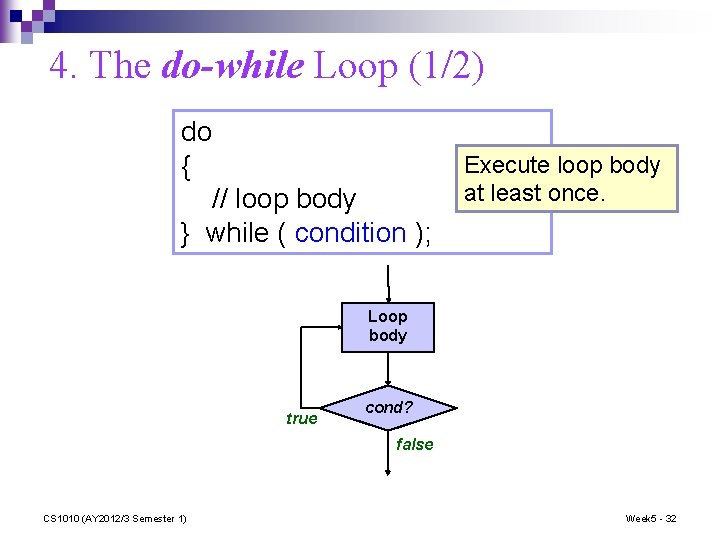4. The do-while Loop (1/2) do { // loop body } while ( condition ); Execute loop body at least once. Loop body true cond? false CS 1010 (AY 2012/3 Semester 1) Week 5 - 32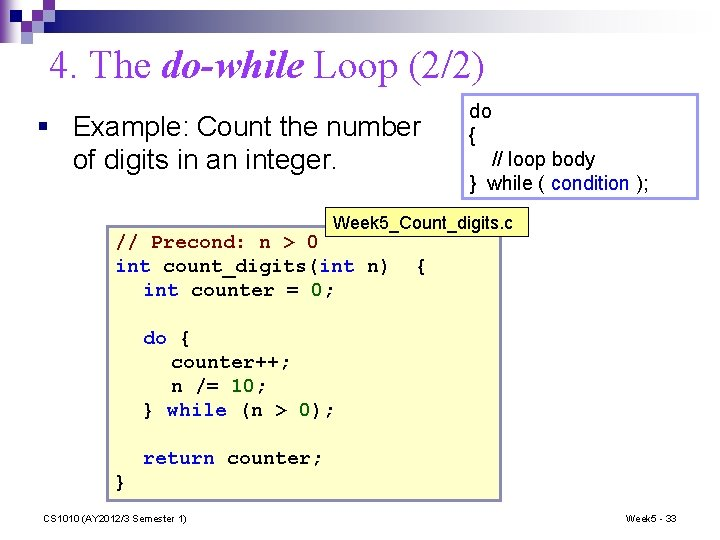4. The do-while Loop (2/2) § Example: Count the number of digits in an integer. do { // loop body } while ( condition ); Week 5_Count_digits. c // Precond: n > 0 int count_digits(int n) int counter = 0; { do { counter++; n /= 10; } while (n > 0); return counter; } CS 1010 (AY 2012/3 Semester 1) Week 5 - 335. The for Loop (1/2) for ( initialization; condition; update ) { // loop body } Initialization: initialize the Condition: repeat loop variable while the condition on loop variable is true Update: change value of loop variable CS 1010 (AY 2012/3 Semester 1) Week 5 - 34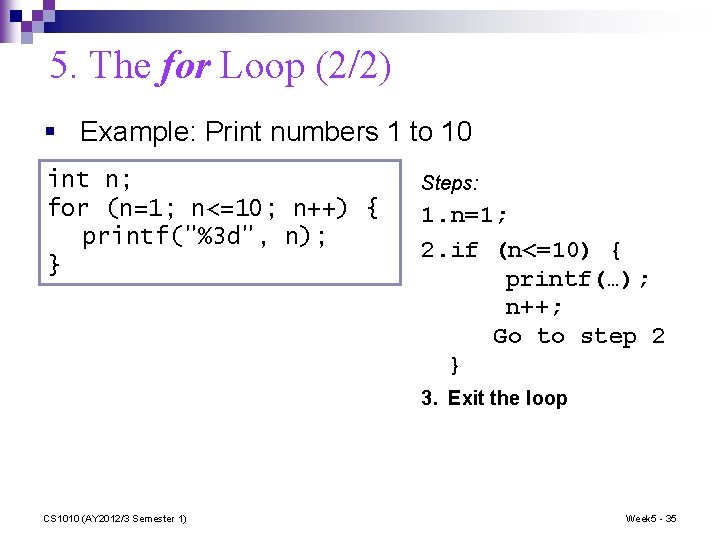5. The for Loop (2/2) § Example: Print numbers 1 to 10 int n; for (n=1; n<=10; n++) { printf("%3 d", n); } Steps: 1. n=1; 2. if (n<=10) { printf(…); n++; Go to step 2 } 3. Exit the loop CS 1010 (AY 2012/3 Semester 1) Week 5 - 355. 1 The for Loop: Odd Integers (1/2) Week 5_Odd. Integers_v 1. c #include <stdio. h> void print_odd_integers(int); int main(void) { int num; printf("Enter a positive integer: "); scanf("%d", &num); print_odd_integers(num); return 0; } // Precond: n > 0 void print_odd_integers(int n) int i; for (i=1; i<=n; i+=2) printf("%d ", i); printf("n"); } CS 1010 (AY 2012/3 Semester 1) { Week 5 - 36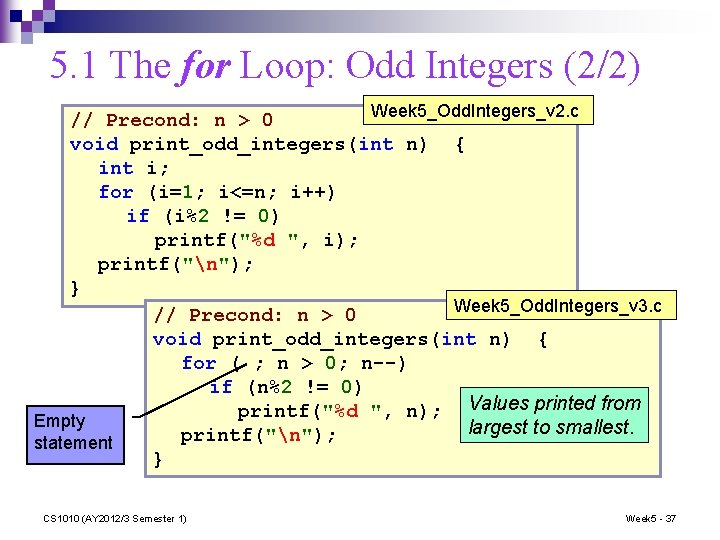5. 1 The for Loop: Odd Integers (2/2) Week 5_Odd. Integers_v 2. c // Precond: n > 0 void print_odd_integers(int n) { int i; for (i=1; i<=n; i++) if (i%2 != 0) printf("%d ", i); printf("n"); } Week 5_Odd. Integers_v 3. c // Precond: n > 0 void print_odd_integers(int n) { for ( ; n > 0; n--) if (n%2 != 0) Values printed from printf("%d ", n); Empty largest to smallest. printf("n"); statement } CS 1010 (AY 2012/3 Semester 1) Week 5 - 37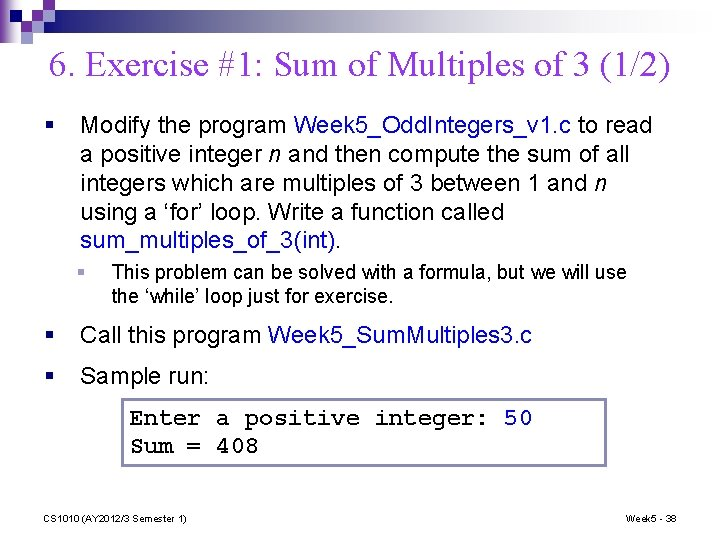6. Exercise #1: Sum of Multiples of 3 (1/2) § Modify the program Week 5_Odd. Integers_v 1. c to read a positive integer n and then compute the sum of all integers which are multiples of 3 between 1 and n using a ‘for’ loop. Write a function called sum_multiples_of_3(int). § This problem can be solved with a formula, but we will use the ‘while’ loop just for exercise. § Call this program Week 5_Sum. Multiples 3. c § Sample run: Enter a positive integer: 50 Sum = 408 CS 1010 (AY 2012/3 Semester 1) Week 5 - 386. Exercise #1: Sum of Multiples of 3 (2/2) § § How about using a while loop instead? Pseudo-code using a while loop: precondition: n > 0 sum 0 i n while i > 0 if i is multiple of 3 then sum + i i i - 1 return sum CS 1010 (AY 2012/3 Semester 1) Week 5 - 397. Exercise #2: Asterisks (1/2) § § § Write a program Week 5_Asterisks. c to read an integer n and print a certain number of asterisks on a single line. Write a function print_asterisks(int). If n is non-positive, then no asterisk should be printed. Sample runs: Think! What is Enter n: 3 ***** Done! Enter n: 6 ****** Done! Enter n: 10 ********** Done! CS 1010 (AY 2012/3 Semester 1) the relationship between n and the number of *? Enter n: -2 Done! Week 5 - 407. Exercise #2: Asterisks (2/2) § Write a program Week 5_Asterisks. c to read an integer n and print a certain number of asterisks on a single line. Write a function print_asterisks(int). Pseudo-code: read input n ; if n is non-positive print “done” and end program ; m compute the number of asterisks given n print_asterisks(m) end program; CS 1010 (AY 2012/3 Semester 1) Week 5 - 41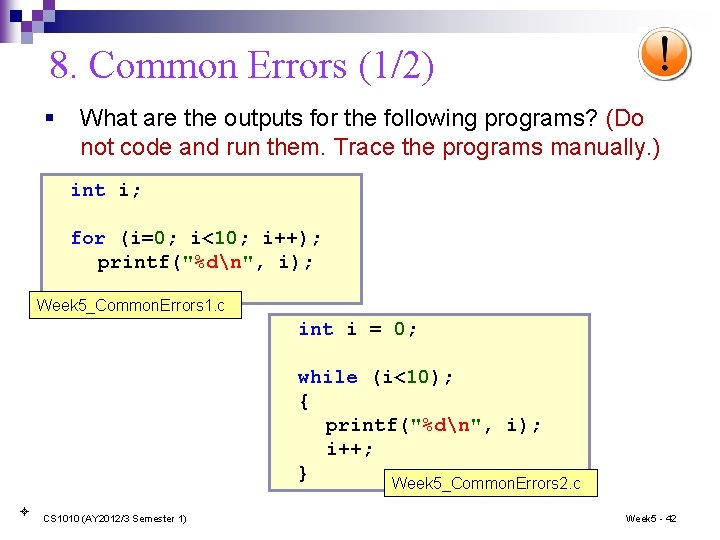8. Common Errors (1/2) § What are the outputs for the following programs? (Do not code and run them. Trace the programs manually. ) int i; for (i=0; i<10; i++); printf("%dn", i); Week 5_Common. Errors 1. c int i = 0; while (i<10); { printf("%dn", i); i++; } Week 5_Common. Errors 2. c CS 1010 (AY 2012/3 Semester 1) Week 5 - 42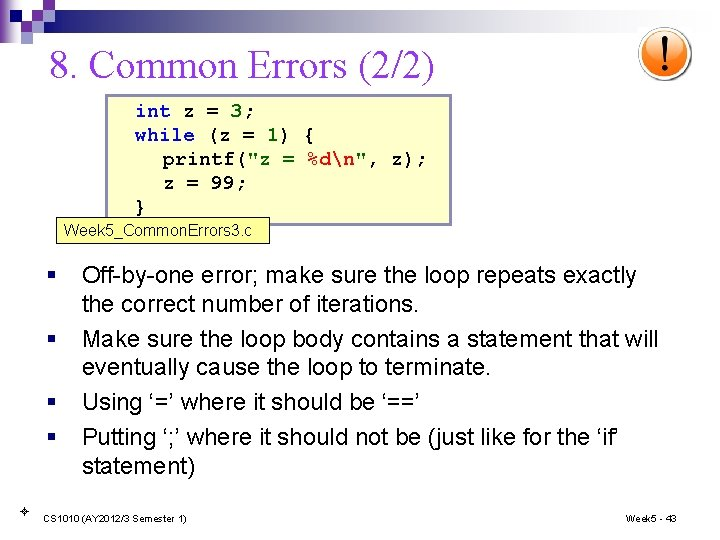8. Common Errors (2/2) int z = 3; while (z = 1) { printf("z = %dn", z); z = 99; } Week 5_Common. Errors 3. c § § Off-by-one error; make sure the loop repeats exactly the correct number of iterations. Make sure the loop body contains a statement that will eventually cause the loop to terminate. Using ‘=’ where it should be ‘==’ Putting ‘; ’ where it should not be (just like for the ‘if’ statement) CS 1010 (AY 2012/3 Semester 1) Week 5 - 43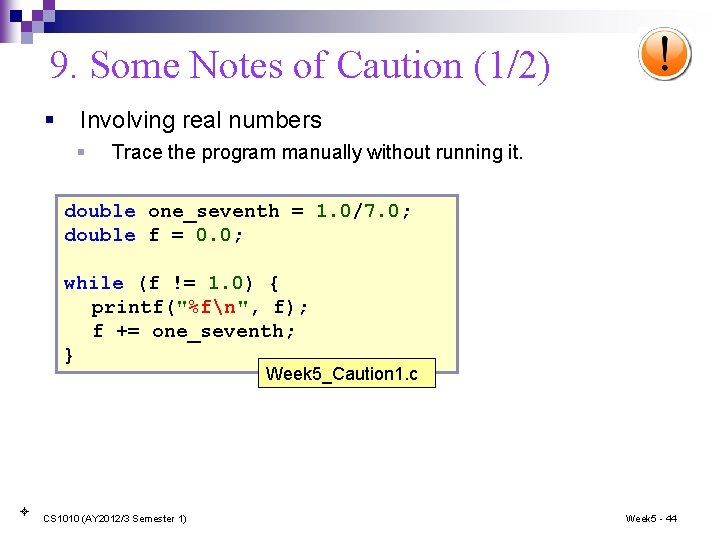9. Some Notes of Caution (1/2) § Involving real numbers § Trace the program manually without running it. double one_seventh = 1. 0/7. 0; double f = 0. 0; while (f != 1. 0) { printf("%fn", f); f += one_seventh; } Week 5_Caution 1. c CS 1010 (AY 2012/3 Semester 1) Week 5 - 44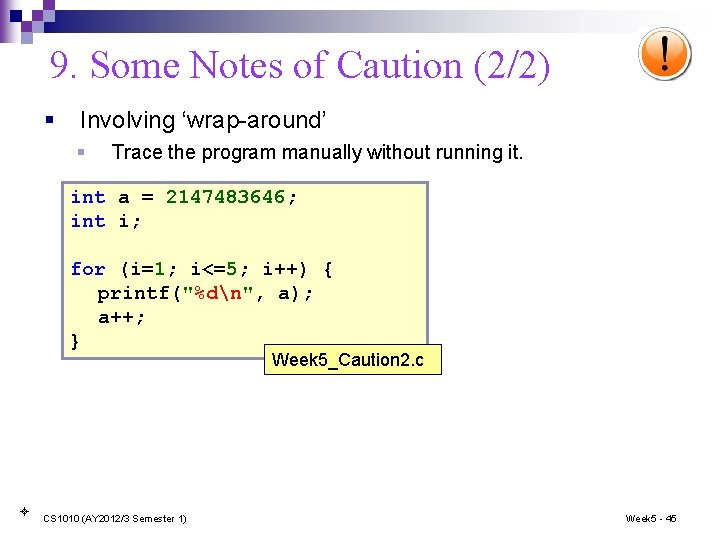9. Some Notes of Caution (2/2) § Involving ‘wrap-around’ § Trace the program manually without running it. int a = 2147483646; int i; for (i=1; i<=5; i++) { printf("%dn", a); a++; } Week 5_Caution 2. c CS 1010 (AY 2012/3 Semester 1) Week 5 - 45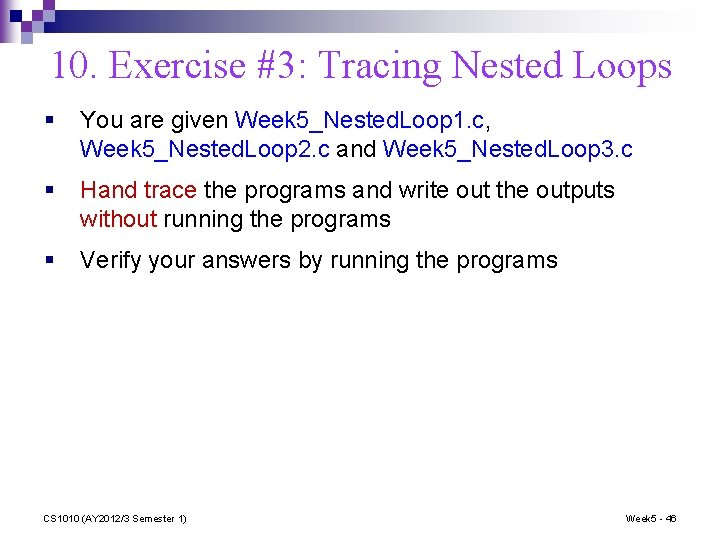10. Exercise #3: Tracing Nested Loops § You are given Week 5_Nested. Loop 1. c, Week 5_Nested. Loop 2. c and Week 5_Nested. Loop 3. c § Hand trace the programs and write out the outputs without running the programs § Verify your answers by running the programs CS 1010 (AY 2012/3 Semester 1) Week 5 - 46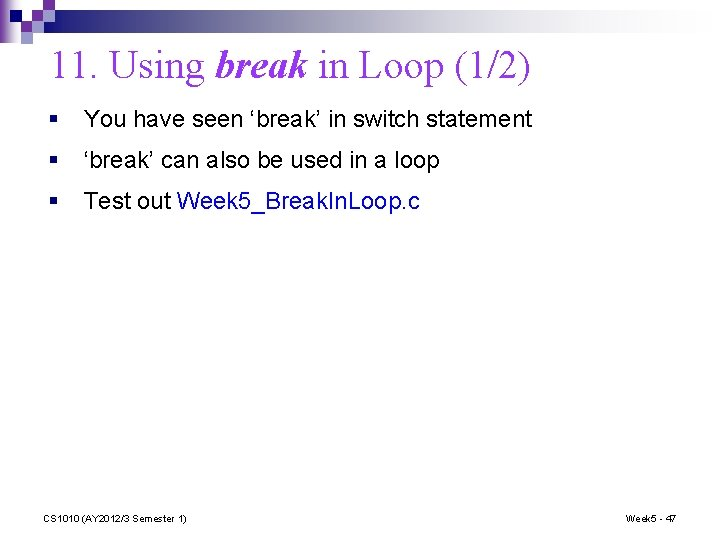11. Using break in Loop (1/2) § You have seen ‘break’ in switch statement § ‘break’ can also be used in a loop § Test out Week 5_Break. In. Loop. c CS 1010 (AY 2012/3 Semester 1) Week 5 - 4711. Using break in Loop (2/2) § § Use ‘break’ sparingly, because it violates the one-entry-one -exit control flow. A loop with ‘break’ can be rewritten into one without ‘break’. // with break int n, i = 1, sum = 0; while (i <= 5) { scanf("%d", &n); if (n < 0) break; sum += n; i++; } CS 1010 (AY 2012/3 Semester 1) // without break int n, i = 1, sum = 0; int is. Valid = 1; while ((i <= 5) && is. Valid){ scanf("%d", &n); if (n < 0) is. Valid = 0; else { sum += n; i++; } } Week 5 - 48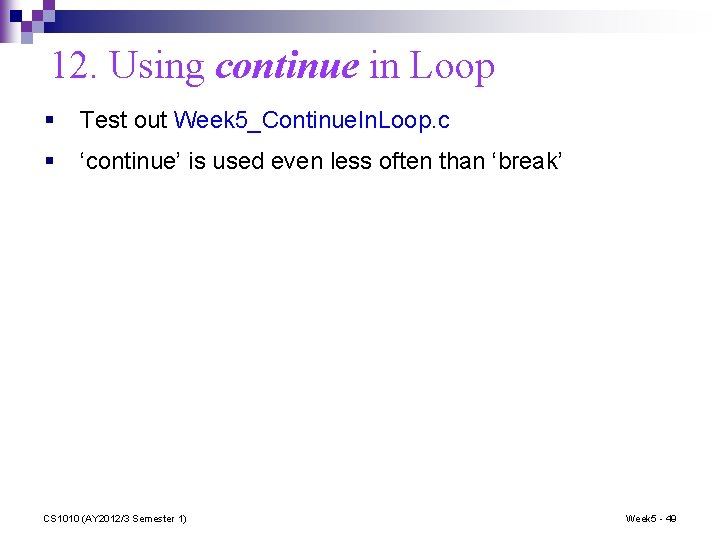12. Using continue in Loop § Test out Week 5_Continue. In. Loop. c § ‘continue’ is used even less often than ‘break’ CS 1010 (AY 2012/3 Semester 1) Week 5 - 4913. Exercise #4: Prime Number (1/2) § Primality test is a classic programming problem § § Given a positive integer, determine whether it is a prime A prime number has two distinct factors (divisors): 1 and itself. Examples: 2, 3, 5, 7, 11, . . . (Note: 1 is not a prime!) § Write a program Week 5_Prime. Test. c. You should include a function is_prime(int). (What does it return? ) § Sample runs: Enter a positive integer: 131 is a prime. Enter a positive integer: 713 is not a prime. CS 1010 (AY 2012/3 Semester 1) Week 5 - 5013. Exercise #4: Prime Number (2/2) § This is your take-home exercise. § This exercise is mounted on Code. Crunch. § We will discuss this in the next lecture. CS 1010 (AY 2012/3 Semester 1) Week 5 - 51Summary for Today (1/2) n Repetition statements (loops) q for, while, do-while q Nested loops q break and continue CS 1010 (AY 2012/3 Semester 1) Week 5 - 52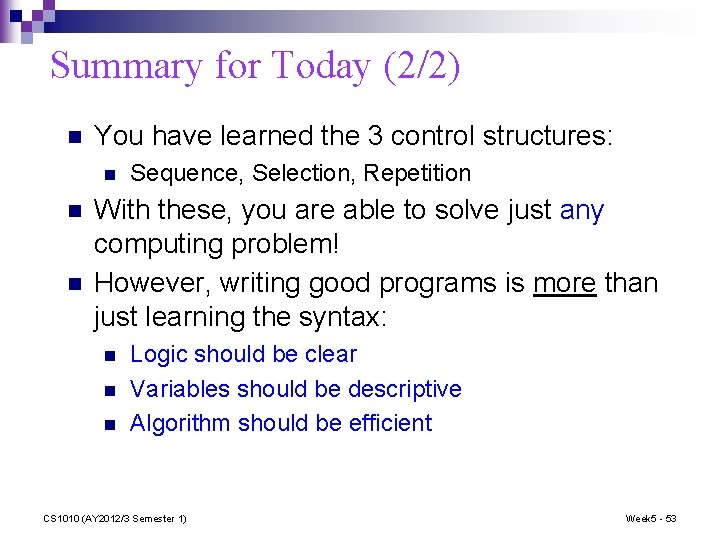Summary for Today (2/2) n You have learned the 3 control structures: n n n Sequence, Selection, Repetition With these, you are able to solve just any computing problem! However, writing good programs is more than just learning the syntax: n n n Logic should be clear Variables should be descriptive Algorithm should be efficient CS 1010 (AY 2012/3 Semester 1) Week 5 - 53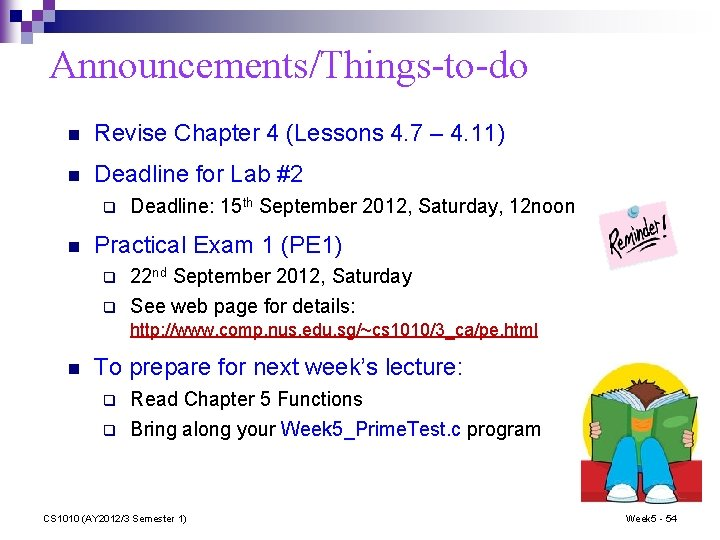Announcements/Things-to-do n Revise Chapter 4 (Lessons 4. 7 – 4. 11) n Deadline for Lab #2 q n Deadline: 15 th September 2012, Saturday, 12 noon Practical Exam 1 (PE 1) q q 22 nd September 2012, Saturday See web page for details: http: //www. comp. nus. edu. sg/~cs 1010/3_ca/pe. html n To prepare for next week’s lecture: q q Read Chapter 5 Functions Bring along your Week 5_Prime. Test. c program CS 1010 (AY 2012/3 Semester 1) Week 5 - 54End of File